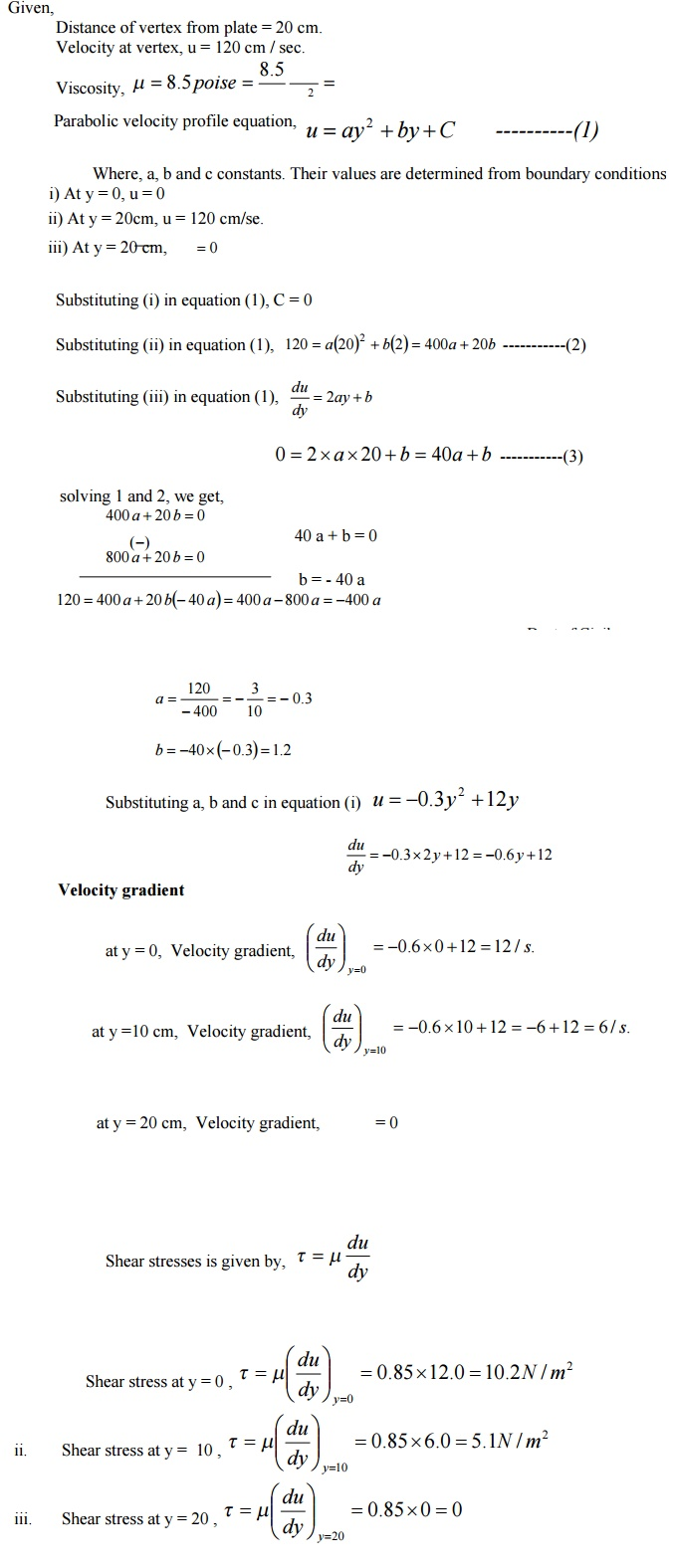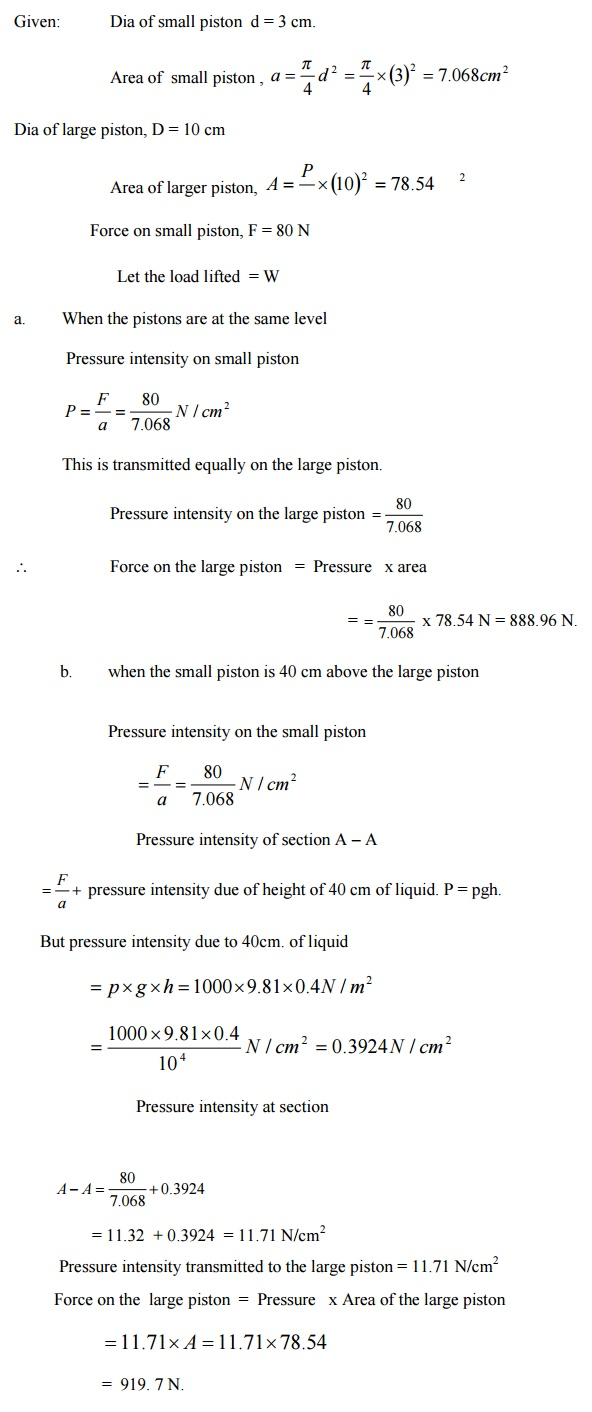Home | | Mechanics of Fluids | Problems: Civil - Fluid Properties

# Problems: Civil - Fluid Properties

Civil - Mechanics Of Fluids - Fluid Properties And Fluid Statics

Problem 1.

Calculate the capillary effect in millimeters a glass tube of 4mm diameter, when immersed in (a) water (b) mercury. The temperature of the liquid is 200 C and the values of the surface tension of water and mercury at 200 C in contact with air are 0.073575 and 0.51 N/m respectively. The angle of contact for water is zero that for mercury 1300. Take specific weight of water as 9790 N / m3Problem 2.

A cylinder of 0.6 m3 in volume contains air at 500C and 0.3 N/ mm2 absolute pressure. The air is compressed to 0.3 m3. Find (i) pressure inside the cylinder assuming isothermal process (ii) pressure and temperature assuming adiabatic process. Take K = 1.4Problem 3

If the velocity profile of a fluid over a plate is a parabolic with the vertex 202 cm from the plate, where the velocity is 120 cm/sec. Calculate the velocity gradients and shear stress at a distance of 0,10 and 20 cm from the plate, if the viscosity of the fluid is 8.5 poise.Problem 4

A 15 cm diameter vertical cylinder rotates concentrically inside another cylinder of diameter 15.10 cm. Both cylinders are 25 cm high. The space between the cylinders is filled with a liquid whose viscosity is unknown. If a torque of 12.0 Nm is required to rotate the inner cylinder at 100 rpm determine the viscosity of the fluid.

Solution:

Diameter of cylinder = 15 cm = 0.15 m

Diameter of outer cylinder = 15.10 cm  = 0.151 m

Length of cylinder  L = 25 cm = 0.25 m

Torque T= 12 Nm ; N = 100 rpm.

Viscosity = mProblem 5

The dynamic viscosity of oil, used for lubrication between a shaft and sleeve is 6 poise. The shaft is of diameter 0.4 m and rotates at 190 rpm. Calculate the power lost in the bearing for a sleeve length of 90 mm. The thickness of the oil film is 1.5 mm.Problem 6

If the velocity distribution over a plate is given by u =2 y/3 -y 2   in which U is the velocity in m/s at a distance y meter above the plate, determine the shear stress at y = 0 and y = 0.15 m. Take dynamic viscosity of fluid as 8.63 poise.Problem 7

The diameters of a small piston and a large piston of a hydraulic jack at3cm and 10 cm respectively. A force of 80 N is applied on the small piston Find the load lifted by the large piston when:

The pistons are at the same level

Small piston in 40 cm above the large piston.

The density of the liquid in the jack in given as 1000 kg/m3

Given:                Dia of small piston  d = 3 cm.Study Material, Lecturing Notes, Assignment, Reference, Wiki description explanation, brief detail
Civil : Mechanics Of Fluids : Fluid Properties And Fluid Statics : Problems: Civil - Fluid Properties |